#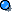The following are the most common types of collision solutions

(note: these examples are from the hyperphysics web site)

## Elastic Collisions - Target Object Initially at Rest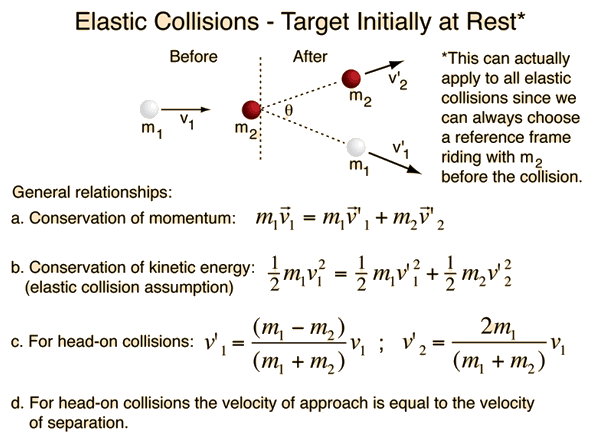Conservation of momentumConservation of kinetic energy## Velocities After Collision

 For head-on elastic collisions , the derived relationship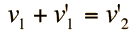may be used along with conservation of momentum equation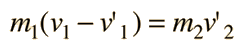to obtain expressions for the individual velocities after the collision.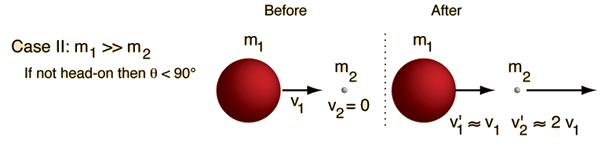# Elastic Collision, Equal Masses

For a head-on collision with a stationary object of equal mass, the projectile will come to rest and the target will move off with equal velocity, like a head-on shot with the cue ball on a pool table. This may be generalized to say that for a head-on elastic collision of equal masses, the velocities will always exchange.For a non-head-on elastic collision between equal masses, the angle between the velocities after the collision will always be 90 degrees. The spot on a pool table is placed so that a collision with a ball on the spot which sends it to a corner pocket will send the cue ball to the other corner pocket.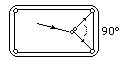# Elastic Collision, Massive Projectile

In a head-on elastic collision where the projectile is much more massive than the target, the velocity of the target particle after the collision will be about twice that of the projectile and the projectile velocity will be essentially unchanged.For non-head-on collisions, the angle between projectile and target is always less than 90 degrees.

This case implies that a train moving at 60 miles/hr which hits a small rock on the track could send that rock forward at 120 miles/hr if the collision were head-on and perfectly elastic. It also implies that if the speed of the head of your golf club is 110 miles/hr, the limiting speed on the golf ball off the tee is 220 miles/hr in the ideal case. That is, the limiting speed of the ball depends strictly on the clubhead speed, and not on how much muscle power you put into the swing.

# Elastic Collision, Massive Target

In a head-on elastic collision between a small projectile and a much more massive target, the projectile will bounce back with essentially the same speed and the massive target will be given a very small velocity. One example is a ball bouncing back from the Earth when we throw it down.In the case of a non-headon elastic collision, the angle of the projectiles path after the collision will be more than 90 degrees away from the targets motion.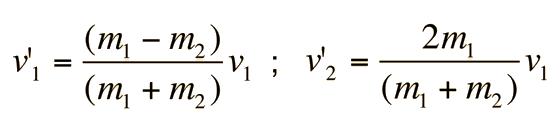can be used to calculate the velocities resulting from any such collision.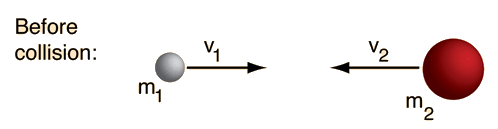Initial conditions: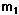=kg,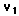=m/s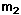=kg,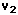=m/s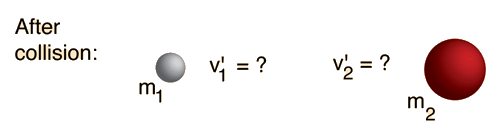Final velocities: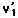= m/s,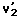= m/s

Velocity of approach = m/s
Velocity of separation = m/s

A positive velocity is taken as a velocity to the right in the calculation, so for leftward motion you should enter a negative number. The calculation assumes that a collision took place, but if you get a negative number for the velocity of approach above, this means you chose a scenario where no collision would actually happen.

In the general case of a one-dimensional collision between two masses, one cannot anticipate how much kinetic energy will be lost in the collision. Therefore, the velocities of the two masses after the collision are not completely determined by their velocities before the collision. However, conservation of momentum must be satisfied, so that if the velocity of one of the particles after the collision is specified, the other is determined.Initial conditions:=kg,=m/s,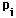=kg m/s=kg,=m/s,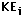=J

Enter values for masses and velocities above. Then specify one final velocity below.Final velocities(specify one, the other will be calculated):= m/s,= m/s
 Final momentum =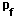=kg m/s, Final kinetic energy =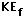J
 For a perfectly elastic collision, the values would have been:= m/s,= m/s

For ordinary objects, the final kinetic energy will be less than the initial value. The only way you can get an increase in kinetic energy is if there is some kind of energy release triggered by the impact. Was one of your objects a hand grenade?

# Inelastic Collision Calculation

Most collisions between objects involve the loss of some kinetic energy and are said to be inelastic. In the general case, the final velocities are not determinable from just the initial velocities. If you know the velocity of one object after the collision, you can determine the other (see inelastic head-on collisions). The extreme inelastic collision is one in which the colliding objects stick together after the collision, and this case may be analyzed in general terms.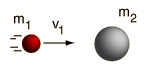Initial data m1 = kg m2 = kg v1 = m/s Momentum = kg m/s Kinetic energy = J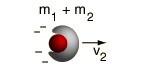Calculated final values   v2 = m/s Momentum = kg m/s Kinetic energy = J

Amount of kinetic energy lost in the collision = J.

 Ratio of kinetic energies before and after the collision: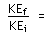Fraction of kinetic energy lost in the collision: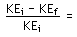# Swinging Balls

A popular demonstration of conservation of momentum and conservation of energy features several polished steel balls hung in a straight line in contact with each other. If one is pulled back and allowed to strike the line, one ball flies out the other end. If two balls are sent in, two come out, and so forth.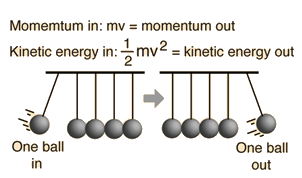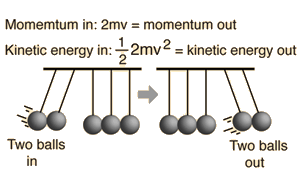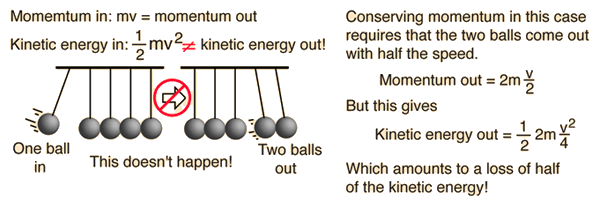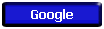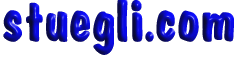Copyright © 2005 -  S. B. EglI